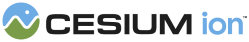#TridiagonalSystemSolver

Uses the Tridiagonal Matrix Algorithm, also known as the Thomas Algorithm, to solve a system of linear equations where the coefficient matrix is a tridiagonal matrix.

### Methods

#### static Cesium.TridiagonalSystemSolver.solve(diagonal, lower, upper, right) → Array.<Cartesian3> engine/Source/Core/TridiagonalSystemSolver.js 43

Solves a tridiagonal system of linear equations.
##### Performance:

Linear time.

Name Type Description
`diagonal` Array.<number> An array with length `n` that contains the diagonal of the coefficient matrix.
`lower` Array.<number> An array with length `n - 1` that contains the lower diagonal of the coefficient matrix.
`upper` Array.<number> An array with length `n - 1` that contains the upper diagonal of the coefficient matrix.
`right` Array.<Cartesian3> An array of Cartesians with length `n` that is the right side of the system of equations.
##### Returns:
An array of Cartesians with length `n` that is the solution to the tridiagonal system of equations.
##### Example:
``````const lowerDiagonal = [1.0, 1.0, 1.0, 1.0];
const diagonal = [2.0, 4.0, 4.0, 4.0, 2.0];
const upperDiagonal = [1.0, 1.0, 1.0, 1.0];
const rightHandSide = [
new Cesium.Cartesian3(410757.0, -1595711.0, 1375302.0),
new Cesium.Cartesian3(-5986705.0, -2190640.0, 1099600.0),
new Cesium.Cartesian3(-12593180.0, 288588.0, -1755549.0),
new Cesium.Cartesian3(-5349898.0, 2457005.0, -2685438.0),
new Cesium.Cartesian3(845820.0, 1573488.0, -1205591.0)
];

const solution = Cesium.TridiagonalSystemSolver.solve(lowerDiagonal, diagonal, upperDiagonal, rightHandSide);``````
Need help? The fastest way to get answers is from the community and team on the Cesium Forum.